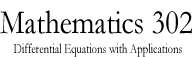Math 302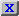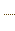HW3.pdfStatement on DisabilitiesStatement on EthicsHomeworkJava ToolsAn example of a circuitAn example of a circuitAn example of a circuitAn Application to Population DynamicsAn application to Population DynamicsSlope Field CalculatorODE 2D CalculatorODE 2D CalculatorODE 3D CalculatorODE 3D CalculatorSlope Field CalculatorSlope Field CalculatorSolution VerifierSolution Verifier 2DSolution Verifier 2DSolution VerifierA Lotke-Volterra SystemA Lotke-Volterra systemLabor Managed Oligopoly - Two firmsODE 3D Calculator2D Map CalculatorA model of sunamiA model of sunamiThe three body problemThe two body problemThe two body problemVan der Pol EquationVan der Pol EquationList of parameters of the JOde AppletBrowser test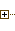Generated Documentation (Untitled)ODE 2D CalculatorUsing Marek Rychliks's Applet for Euler's MethodJOde Manual PageJOde - An Applet for Studying Ordinary Differential Equations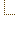ODE 2D CalculatorSyllabus
 Math Dept. Home Help room
Parameter Value type Description
label String Label string to be displayed at the top of the applet
editable boolean If true, the applet allows editing equations, initial conditions, etc.
background String Background color, format "rrggbb"
foreground String Foreground color, format "rrggbb"
dimension int Number of ODE's
parameter String Parameter (indep. variable) of ODE's, same as variable0
variableN String Name of variable #N, N=0,1,..., dimension (variable0 is the indep. variable)
equationN String Expression of equation #N, N=0,...,dimension; equation0 is synonymous with "initcode".
functionN String Expression of function #N, N=0,...,dimension
initcode String Expression of equation #0; this expression is evaluated only once; can be used to define constants, functions, etc.
minN double/expression Low limit for variableN, N=0,1,...,dimension
maxN double/expression High limit for variableN, N=0,1,...,dimension
segmentsN int Number of segments in the grid for variable #N
parametersegments int Number of segments in the grid of the parameter, i.e. variable0
initconds String List of init. coonditions, e.g "0,0;1.2,1;1,2" or "(0,0),(1.2,1),(1,2)"
showslopes boolean If set, display slope field
showtickmarks boolean If set, display tick marks around the plot
showsolutions boolean If set, display solutions specified by initconds
showfunctions boolean If set, display graphs of functions
showequations boolean If set, display equations
showranges boolean If set, display ranges of variables and axis selection tools near the border of the phase diagram
showinitconditions boolean If set, display and label init. conditions
showlines boolean If set, show segments of lines joining two consecutive calculated points in solutions
showpoints boolean If set, show calculated points of solutions
algorithm String Sets the numerical algorithm. Known: Euler, ModifiedEuler, Midpoint, RK4 (Runge-Kutta 4), RKF (Runge-Kutta-Fehlberg)
isframed boolean If set, the applet runs in a frame.
autonomous boolean If set, the system is autonomous, the paramater (variable0) does not appear in ODE's
minparameter double/expression Low limit for the time parameter
maxparameter double/expression High limit for the time parameter
minx double Low end of x-range (when dim=2, autonomous=false only)
maxx double High end of x-range (dim=2, autonomous=false only)
miny double Low end of y-range (dim=2, autonomous=false only)
maxy double High end of y-range (dim=2, autonomous=false only)
formula String Right-hand side of ODE y'=f(x,y) (dim=2, autonomous=false only)
xsegments int Number of segments in the x-direction (dim=2, autonomous=false only)
ysegments int Number of segments in the y-direction (dim=2, autonomous=false only)
abscissa int Index of variable to be used as abscissa of the plot (default 0 if autonomous = false, 1 otherwise).
ordinate int Index of variable to be used as ordinate of the plot (default 1 if autonomous = false, 2 otherwise).
step double Step of integration.
nStepsLimit int Maximal number of steps (vector field of function evaluations) to obtain a single curve (default: 100000).
librarycode String Library of code which will be evaluated once when the applet is started.
lefthanded boolean If true, some controls will appear on the left, instead of right.
options String A sequence of parameter=value assignments with ";" as separator, e.g. "dimension = 2; isframed = true". Trailing blanks and tabs in value and around "=" are discarded. This option is an alternative to all other options, and is espectially convenient if the settings to the applet are generated automatically by running an external program.